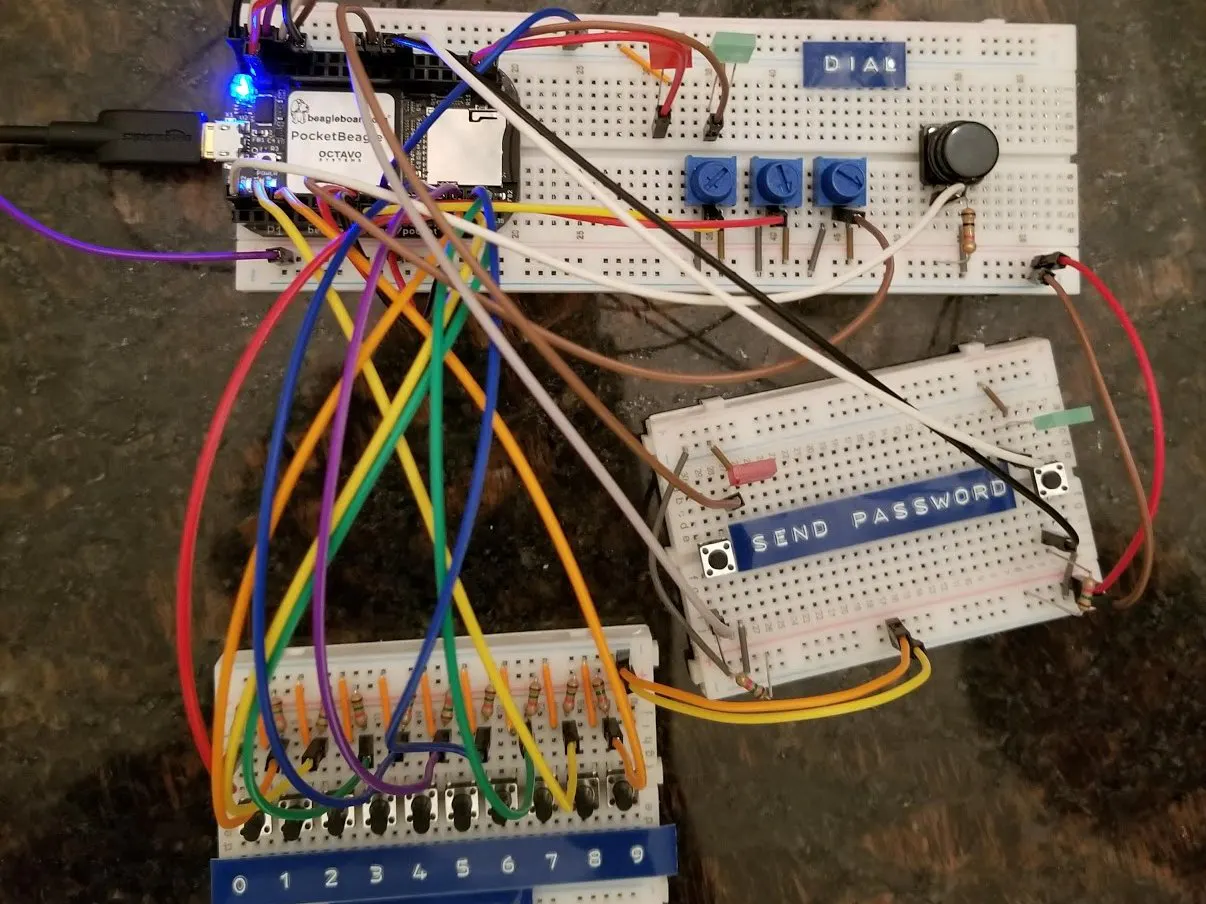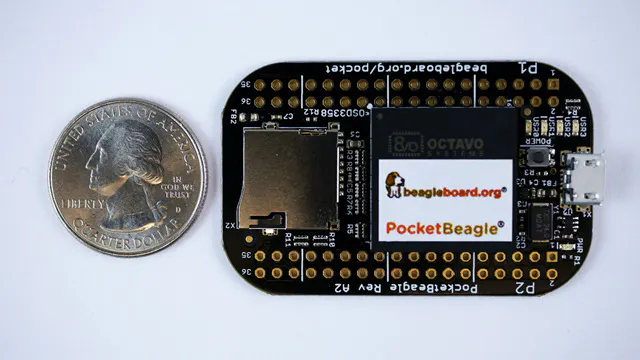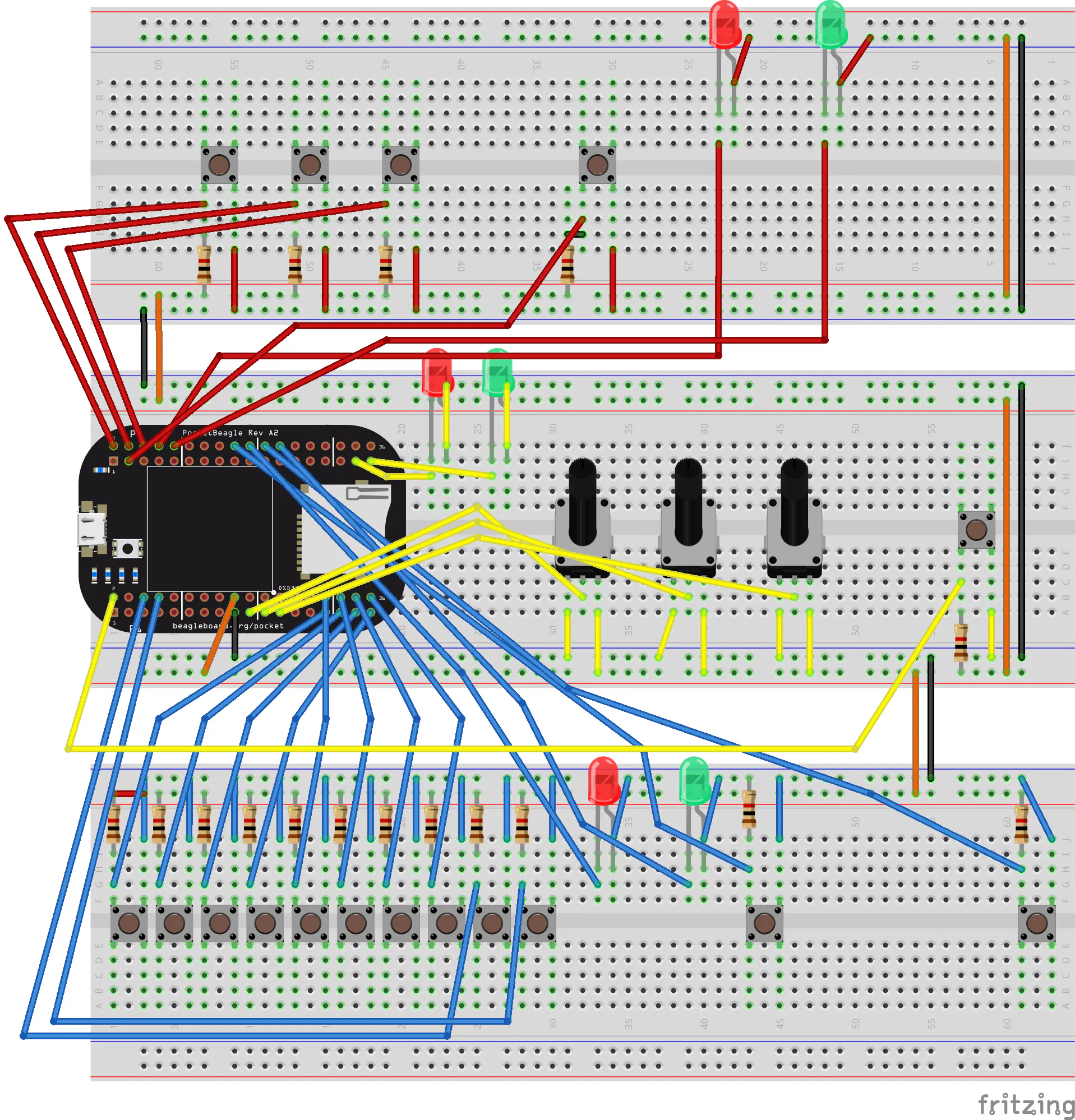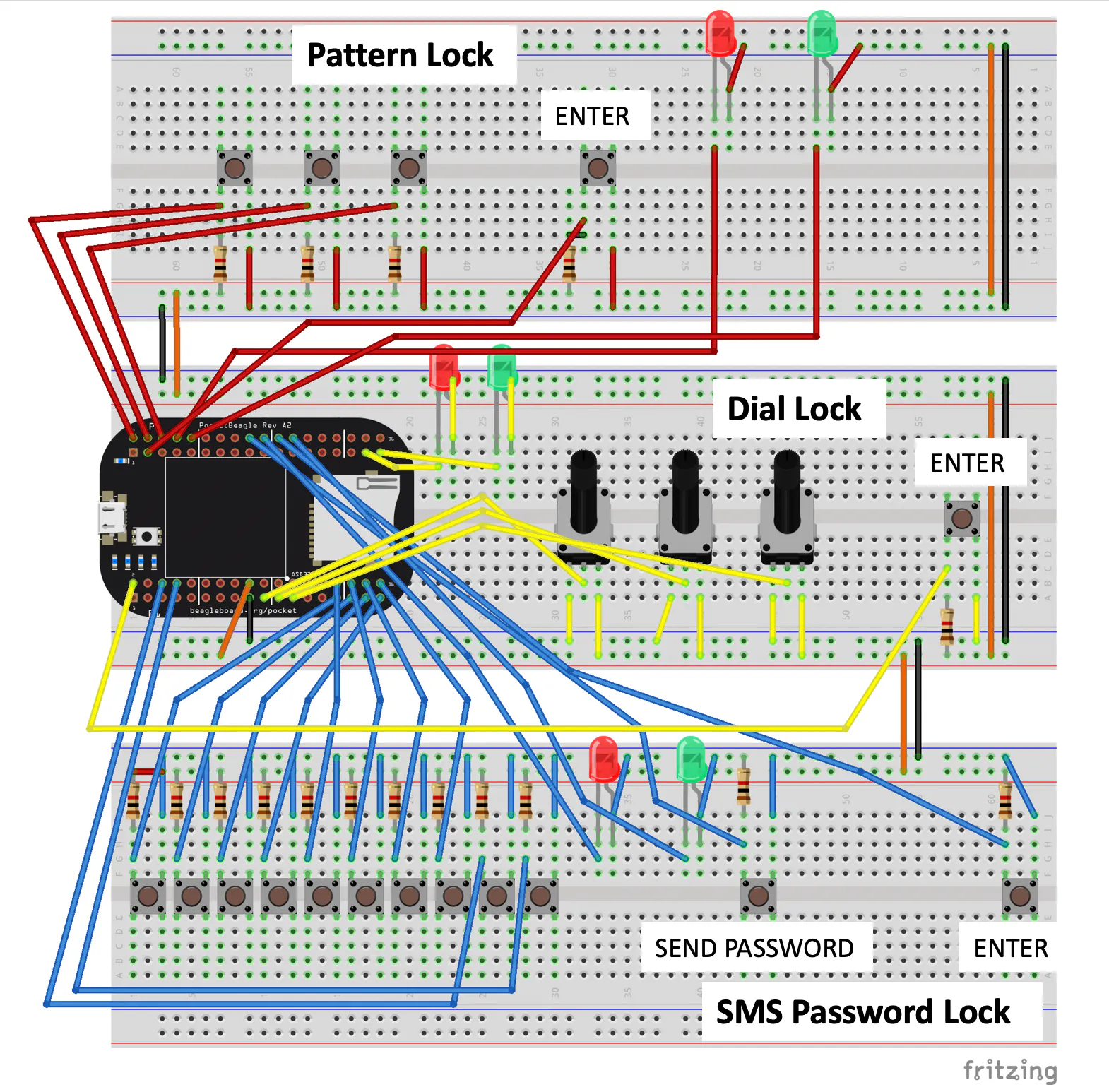Published

# The "Stupidly Secure" Safe (with Twilio 2FA)

Create a prototype security system with three sequential layers of security -- a dial lock, pattern lock, and keypad password!

IntermediateFull instructions provided10 hours450## Things used in this project

### Hardware componentsBeagleBoard.org PocketBeagle
×1Jumper wires (generic)
×15 mm LED: Red
×35 mm LED: Green
×3Rotary potentiometer (generic)
×3Resistor 1k ohm
×17SparkFun Pushbutton switch 12mm
×17

### Software apps and online servicesTwilio SMS Messaging API

## Schematics

### Unlabeled Fritzing

An unlabeled fritzing diagram of the project### Labeled Fritzing Diagram

Fritzing diagram labeled with extra button functionalities and breadboard labels## Code

### Project_Dials_v8.py

Python
Main project script
```# -*- coding: utf-8 -*-
"""
--------------------------------------------------------------------------
Create a 3 layer security system with various password locks
--------------------------------------------------------------------------

Redistribution and use in source and binary forms, with or without
modification, are permitted provided that the following conditions are met:

1. Redistributions of source code must retain the above copyright notice,
this list of conditions and the following disclaimer.

2. Redistributions in binary form must reproduce the above copyright notice,
this list of conditions and the following disclaimer in the documentation
and/or other materials provided with the distribution.

3. Neither the name of the copyright holder nor the names of its contributors
may be used to endorse or promote products derived from this software without
specific prior written permission.

THIS SOFTWARE IS PROVIDED BY THE COPYRIGHT HOLDERS AND CONTRIBUTORS "AS IS"
AND ANY EXPRESS OR IMPLIED WARRANTIES, INCLUDING, BUT NOT LIMITED TO, THE
IMPLIED WARRANTIES OF MERCHANTABILITY AND FITNESS FOR A PARTICULAR PURPOSE ARE
DISCLAIMED. IN NO EVENT SHALL THE COPYRIGHT HOLDER OR CONTRIBUTORS BE LIABLE
FOR ANY DIRECT, INDIRECT, INCIDENTAL, SPECIAL, EXEMPLARY, OR CONSEQUENTIAL
DAMAGES (INCLUDING, BUT NOT LIMITED TO, PROCUREMENT OF SUBSTITUTE GOODS OR
SERVICES; LOSS OF USE, DATA, OR PROFITS; OR BUSINESS INTERRUPTION) HOWEVER
CAUSED AND ON ANY THEORY OF LIABILITY, WHETHER IN CONTRACT, STRICT LIABILITY,
OR TORT (INCLUDING NEGLIGENCE OR OTHERWISE) ARISING IN ANY WAY OUT OF THE USE
OF THIS SOFTWARE, EVEN IF ADVISED OF THE POSSIBILITY OF SUCH DAMAGE.
--------------------------------------------------------------------------

Code Structure
- Import Libraries
- Define inputs and outputs
- Define global variables
- Pattern lock function
- SMS + Keypad lock function
- Main Script

--------------------------------------------------------------------------

"""
import time

from twilio.rest import Client

# Import random to generate random number code
from random import randint

# Import date to use date in text message
from datetime import datetime

# ------------------------------------------------------------------------
# Constants
# ------------------------------------------------------------------------

"""
config-pin P1_29 gpio
config-pin P1_31 gpio
config-pin P1_33 gpio
config-pin P1_35 gpio

config-pin P1_30 gpio
config-pin P1_32 gpio
config-pin P1_34 gpio
config-pin P1_36 gpio

config-pin P1_6 gpio
config-pin P1_8 gpio
"""

# Three-Dial lock
ANALOG_INPUT1 = "P1_19"
ANALOG_INPUT2 = "P1_21"
ANALOG_INPUT3 = "P1_23"

INCORRECT_OUTPUT = "P2_33"
CORRECT_OUTPUT = "P2_35"

BUTTON0 = "P1_2"

# Pattern combo lock
PATTERN_BUTTON1 = "P2_2"
PATTERN_BUTTON2 = "P2_4"
PATTERN_BUTTON3 = "P2_6"

PATTERN_INCORRECT_OUTPUT = "P2_8"
PATTERN_CORRECT_OUTPUT = "P2_10"

PATTERN_ENTER_BUTTON = "P2_3"

SMS_SEND_BUTTON = "P2_18"
SMS_ENTER_BUTTON = "P2_22"

SMS_INCORRECT_OUTPUT = "P2_20"
SMS_CORRECT_OUTPUT = "P2_24"

SMS_BUTTON_0 = "P1_29"
SMS_BUTTON_1 = "P1_31"
SMS_BUTTON_2 = "P1_33"
SMS_BUTTON_3 = "P1_35"
SMS_BUTTON_4 = "P1_30"
SMS_BUTTON_5 = "P1_32"
SMS_BUTTON_6 = "P1_34"
SMS_BUTTON_7 = "P1_36"
SMS_BUTTON_8 = "P1_6"
SMS_BUTTON_9 = "P1_8"

# ------------------------------------------------------------------------
# Global variables
# ------------------------------------------------------------------------
debug = True

DIAL_CORRECT_VALUE = 0

PATTERN_CORRECT_VALUE = 0

SMS_CORRECT_VALUE= 0

# ------------------------------------------------------------------------
# Functions
# ------------------------------------------------------------------------

def setup():

# DIAL --------------
# Output
GPIO.setup(INCORRECT_OUTPUT, GPIO.OUT)
GPIO.setup(CORRECT_OUTPUT, GPIO.OUT)

# Button input
GPIO.setup(BUTTON0, GPIO.IN)

# PATTERN --------------
# Button Inputs
GPIO.setup(PATTERN_BUTTON1, GPIO.IN)
GPIO.setup(PATTERN_BUTTON2, GPIO.IN)
GPIO.setup(PATTERN_BUTTON3, GPIO.IN)
GPIO.setup(PATTERN_ENTER_BUTTON, GPIO.IN)

#Outputs
GPIO.setup(PATTERN_CORRECT_OUTPUT, GPIO.OUT)
GPIO.setup(PATTERN_INCORRECT_OUTPUT, GPIO.OUT)

# Button Inputs
GPIO.setup(SMS_SEND_BUTTON, GPIO.IN)
GPIO.setup(SMS_ENTER_BUTTON, GPIO.IN)

GPIO.setup(SMS_BUTTON_0, GPIO.IN)
GPIO.setup(SMS_BUTTON_1, GPIO.IN)
GPIO.setup(SMS_BUTTON_2, GPIO.IN)
GPIO.setup(SMS_BUTTON_3, GPIO.IN)
GPIO.setup(SMS_BUTTON_4, GPIO.IN)
GPIO.setup(SMS_BUTTON_5, GPIO.IN)
GPIO.setup(SMS_BUTTON_6, GPIO.IN)
GPIO.setup(SMS_BUTTON_7, GPIO.IN)
GPIO.setup(SMS_BUTTON_8, GPIO.IN)
GPIO.setup(SMS_BUTTON_9, GPIO.IN)

#Outputs
GPIO.setup(SMS_CORRECT_OUTPUT, GPIO.OUT)
GPIO.setup(SMS_INCORRECT_OUTPUT, GPIO.OUT)

global DIAL_CORRECT_VALUE
DIAL_CORRECT_VALUE = 0

while(GPIO.input(BUTTON0) == 1):
pass

# Gets angle from potentiometers

if (angle1 < float(0.5) and angle2 < float(0.5) and angle3 < float(0.5)):
if (debug): print("Dial: Correct")
GPIO.output(CORRECT_OUTPUT, GPIO.HIGH)
GPIO.output(INCORRECT_OUTPUT, GPIO.LOW)

DIAL_CORRECT_VALUE = 1

else:
GPIO.output(CORRECT_OUTPUT, GPIO.LOW)
GPIO.output(INCORRECT_OUTPUT, GPIO.HIGH)
if (debug): print("Dial: Wrong Combo")
time.sleep(1)
GPIO.output(INCORRECT_OUTPUT, GPIO.LOW)

if (debug): print(DIAL_CORRECT_VALUE)

while(GPIO.input(BUTTON0) == 0):
pass

global PATTERN_CORRECT_VALUE
PATTERN_CORRECT_VALUE = 0;

time.sleep(0.2)

if (debug):
print(GPIO.input(PATTERN_BUTTON1))
print(GPIO.input(PATTERN_BUTTON2))
print(GPIO.input(PATTERN_BUTTON3))

# The current password (1, 2, 1, 1) is currently hardcoded
# Checks if correct button is pressed then moves on in script
while(GPIO.input(PATTERN_BUTTON1) == 1 and GPIO.input(PATTERN_BUTTON2) == 1 and GPIO.input(PATTERN_BUTTON3) == 1):
pass

if (GPIO.input(PATTERN_BUTTON1) == 0 and GPIO.input(PATTERN_BUTTON2) == 1 and GPIO.input(PATTERN_BUTTON3) == 1):
if (debug): print("Pattern: step one complete")

time.sleep(0.2)
while(GPIO.input(PATTERN_BUTTON1) == 1 and GPIO.input(PATTERN_BUTTON2) == 1 and GPIO.input(PATTERN_BUTTON3) == 1):
pass

if (GPIO.input(PATTERN_BUTTON2) == 0 and GPIO.input(PATTERN_BUTTON1) == 1 and GPIO.input(PATTERN_BUTTON3) == 1):
if (debug): print("Pattern: set two complete")

time.sleep(0.2)
while(GPIO.input(PATTERN_BUTTON1) == 1 and GPIO.input(PATTERN_BUTTON2) == 1 and GPIO.input(PATTERN_BUTTON3) == 1):
pass

if (GPIO.input(PATTERN_BUTTON1) == 0 and GPIO.input(PATTERN_BUTTON2) == 1 and GPIO.input(PATTERN_BUTTON3) == 1):
if (debug): print("Pattern: set three complete")

time.sleep(0.2)
while(GPIO.input(PATTERN_BUTTON1) == 1 and GPIO.input(PATTERN_BUTTON2) == 1 and GPIO.input(PATTERN_BUTTON3) == 1):
pass

if (GPIO.input(PATTERN_BUTTON1) == 0 and GPIO.input(PATTERN_BUTTON2) == 1 and GPIO.input(PATTERN_BUTTON3) == 1):
if (debug): print("Pattern: set four complete")

PATTERN_CORRECT_VALUE = 1;

else:
if (debug): print("Pattern: Failure (4)")
else:
if (debug): print("Pattern: Failure (3)")
else:
if (debug): print("Pattern: Failure (2)")
else:
if (debug): print("Pattern: Failure (1)")

while(GPIO.input(PATTERN_ENTER_BUTTON) == 1):
pass

if(PATTERN_CORRECT_VALUE == 1):
if (debug): print("Pattern: Combination complete")
GPIO.output(PATTERN_CORRECT_OUTPUT, GPIO.HIGH)
GPIO.output(PATTERN_INCORRECT_OUTPUT, GPIO.LOW)
else:
if (debug): print("Pattern: wrong combo, resetting")
GPIO.output(PATTERN_CORRECT_OUTPUT, GPIO.LOW)
GPIO.output(PATTERN_INCORRECT_OUTPUT, GPIO.HIGH)
time.sleep(1)
GPIO.output(PATTERN_INCORRECT_OUTPUT, GPIO.LOW)

while(GPIO.input(BUTTON0) == 0):
pass

global SMS_CORRECT_VALUE
SMS_CORRECT_VALUE = 0

# Create random code

SMS_Button_Array = [SMS_BUTTON_0, SMS_BUTTON_1, SMS_BUTTON_2, SMS_BUTTON_3, SMS_BUTTON_4, SMS_BUTTON_5, SMS_BUTTON_6, SMS_BUTTON_7, SMS_BUTTON_8, SMS_BUTTON_9]

# datetime object containing current date and time
now = datetime.now()

dt_string = now.strftime("%Y-%m-%d %H:%M:%S")

# Wait until "SEND" button is pressed
while(GPIO.input(SMS_SEND_BUTTON) == 1):
pass

# Your Account Sid and Auth Token from twilio.com/console
# DANGER! This is insecure. See http://twil.io/secure
# CENSORED FOR HACKSTER.IO
account_sid = 'xxxxxxxxxx'
auth_token = 'xxxxxxxxxxx'
client = Client(account_sid, auth_token)

# NUMBERS CENSORED FOR HACKSTER.IO
message = client.messages \
.create(
from_='xxxxxxxxxxx',
to='xxxxxxxxxx'
)

if (debug):
print(message.body)

# In similar fashion to pattern, check to see if 1st button is pressed corrected then moved on
# Code is formatted to handle any 5-digit password generated
while (SMS_CORRECT_VALUE == 0):

while(GPIO.input(SMS_BUTTON_0) == 0 and GPIO.input(SMS_BUTTON_1) == 0 and GPIO.input(SMS_BUTTON_2) == 0 and GPIO.input(SMS_BUTTON_3) == 0 and GPIO.input(SMS_BUTTON_4) == 0 and GPIO.input(SMS_BUTTON_5) == 0 and GPIO.input(SMS_BUTTON_6) == 0 and GPIO.input(SMS_BUTTON_7) == 0 and GPIO.input(SMS_BUTTON_8) == 0 and GPIO.input(SMS_BUTTON_9) == 0):
pass

if (debug): print("SMS: step one complete")

time.sleep(0.2)
while(GPIO.input(SMS_BUTTON_0) == 0 and GPIO.input(SMS_BUTTON_1) == 0 and GPIO.input(SMS_BUTTON_2) == 0 and GPIO.input(SMS_BUTTON_3) == 0 and GPIO.input(SMS_BUTTON_4) == 0 and GPIO.input(SMS_BUTTON_5) == 0 and GPIO.input(SMS_BUTTON_6) == 0 and GPIO.input(SMS_BUTTON_7) == 0 and GPIO.input(SMS_BUTTON_8) == 0 and GPIO.input(SMS_BUTTON_9) == 0):
pass

if (debug):print("SMS: set two complete")

time.sleep(0.2)
while(GPIO.input(SMS_BUTTON_0) == 0 and GPIO.input(SMS_BUTTON_1) == 0 and GPIO.input(SMS_BUTTON_2) == 0 and GPIO.input(SMS_BUTTON_3) == 0 and GPIO.input(SMS_BUTTON_4) == 0 and GPIO.input(SMS_BUTTON_5) == 0 and GPIO.input(SMS_BUTTON_6) == 0 and GPIO.input(SMS_BUTTON_7) == 0 and GPIO.input(SMS_BUTTON_8) == 0 and GPIO.input(SMS_BUTTON_9) == 0):
pass

if (debug):print("SMS: set three complete")

time.sleep(0.2)
while(GPIO.input(SMS_BUTTON_0) == 0 and GPIO.input(SMS_BUTTON_1) == 0 and GPIO.input(SMS_BUTTON_2) == 0 and GPIO.input(SMS_BUTTON_3) == 0 and GPIO.input(SMS_BUTTON_4) == 0 and GPIO.input(SMS_BUTTON_5) == 0 and GPIO.input(SMS_BUTTON_6) == 0 and GPIO.input(SMS_BUTTON_7) == 0 and GPIO.input(SMS_BUTTON_8) == 0 and GPIO.input(SMS_BUTTON_9) == 0):
pass

if (debug):print("SMS: set four complete")

time.sleep(0.2)
while(GPIO.input(SMS_BUTTON_0) == 0 and GPIO.input(SMS_BUTTON_1) == 0 and GPIO.input(SMS_BUTTON_2) == 0 and GPIO.input(SMS_BUTTON_3) == 0 and GPIO.input(SMS_BUTTON_4) == 0 and GPIO.input(SMS_BUTTON_5) == 0 and GPIO.input(SMS_BUTTON_6) == 0 and GPIO.input(SMS_BUTTON_7) == 0 and GPIO.input(SMS_BUTTON_8) == 0 and GPIO.input(SMS_BUTTON_9) == 0):
pass

if (debug): print("SMS: set five complete")

SMS_CORRECT_VALUE = 1;

else:
if (debug): print("SMS: Failure (5)")
else:
if (debug): print("SMS: Failure (4)")
else:
if (debug): print("SMS: Failure (3)")
else:
if (debug): print("SMS: Failure (2)")
else:
if (debug): print("SMS: Failure (1)")

while(GPIO.input(SMS_ENTER_BUTTON) == 1):
pass

if(SMS_CORRECT_VALUE == 1):
if (debug): print("SMS: Correct combination")
GPIO.output(SMS_CORRECT_OUTPUT, GPIO.HIGH)
GPIO.output(SMS_INCORRECT_OUTPUT, GPIO.LOW)
else:
if (debug): print("SMS: Wrong Combination, resetting")
GPIO.output(SMS_CORRECT_OUTPUT, GPIO.LOW)
GPIO.output(SMS_INCORRECT_OUTPUT, GPIO.HIGH)
time.sleep(1)
GPIO.output(SMS_INCORRECT_OUTPUT, GPIO.LOW)

# ------------------------------------------------------------------------
# Main script
# ------------------------------------------------------------------------

if __name__ == '__main__':

setup()

# Runs through 3-layer protocol
# Note that these have to be done in order! This adds to the secure aspect of the system
print("Begin safe security protocol")

while (DIAL_CORRECT_VALUE == 0):

while (PATTERN_CORRECT_VALUE == 0):

print("End security safe protocol -- Unlocking door now...")
```

### Safe_Run.sh

SH
Shell script to run main project code
```# !/bin/bash
"""
--------------------------------------------------------------------------
RUN: Create a 3 layer security system with various password locks
--------------------------------------------------------------------------

Redistribution and use in source and binary forms, with or without
modification, are permitted provided that the following conditions are met:

1. Redistributions of source code must retain the above copyright notice,
this list of conditions and the following disclaimer.

2. Redistributions in binary form must reproduce the above copyright notice,
this list of conditions and the following disclaimer in the documentation
and/or other materials provided with the distribution.

3. Neither the name of the copyright holder nor the names of its contributors
may be used to endorse or promote products derived from this software without
specific prior written permission.

THIS SOFTWARE IS PROVIDED BY THE COPYRIGHT HOLDERS AND CONTRIBUTORS "AS IS"
AND ANY EXPRESS OR IMPLIED WARRANTIES, INCLUDING, BUT NOT LIMITED TO, THE
IMPLIED WARRANTIES OF MERCHANTABILITY AND FITNESS FOR A PARTICULAR PURPOSE ARE
DISCLAIMED. IN NO EVENT SHALL THE COPYRIGHT HOLDER OR CONTRIBUTORS BE LIABLE
FOR ANY DIRECT, INDIRECT, INCIDENTAL, SPECIAL, EXEMPLARY, OR CONSEQUENTIAL
DAMAGES (INCLUDING, BUT NOT LIMITED TO, PROCUREMENT OF SUBSTITUTE GOODS OR
SERVICES; LOSS OF USE, DATA, OR PROFITS; OR BUSINESS INTERRUPTION) HOWEVER
CAUSED AND ON ANY THEORY OF LIABILITY, WHETHER IN CONTRACT, STRICT LIABILITY,
OR TORT (INCLUDING NEGLIGENCE OR OTHERWISE) ARISING IN ANY WAY OUT OF THE USE
OF THIS SOFTWARE, EVEN IF ADVISED OF THE POSSIBILITY OF SUCH DAMAGE.
--------------------------------------------------------------------------

Code Structure
- Set pin functions
- Run python code

--------------------------------------------------------------------------

"""

cd /var/lib/cloud9/ENGI301/python

config-pin P1_29 gpio
config-pin P1_31 gpio
config-pin P1_33 gpio
config-pin P1_35 gpio

config-pin P1_30 gpio
config-pin P1_32 gpio
config-pin P1_34 gpio
config-pin P1_36 gpio

config-pin P1_6 gpio
config-pin P1_8 gpio

python3 Project_Dials_v8.py

@reboot sleep 30 && sh /var/lib/cloud9/ENGI301/python/Project_Dials_v8.py > /var/lib/cloud9/cronlog 2>&1
```

## Credits

### John Perez

1 project • 0 followers